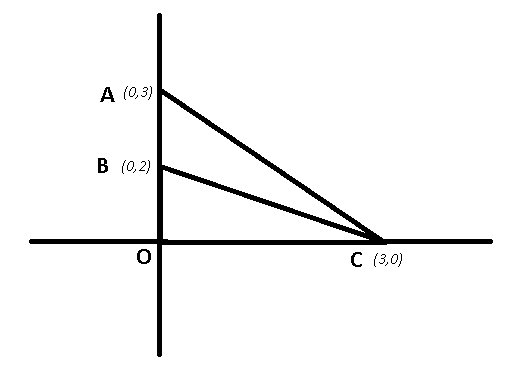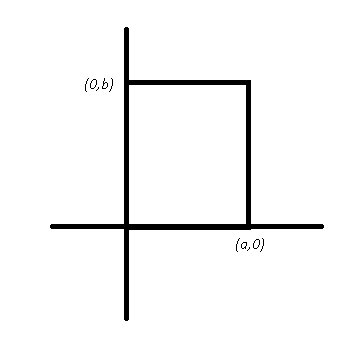0
741

Questions on Linear Equations For SSC CGL Exam

Download the most important Linear Equations questions for SSC CGL exam 2020. Most important linear equation questions based on asked questions in previous exam papers for SSC CGL Exam. These questions are really helpful to crack the SSC exams and all competitive exams.

Question 1: A point in the 4th quadrant is 6 unit away from x-axis and 7 unit away from y-axis. The point is at

a) (7, -6)

b) (-7, 6)

c) (-6, -7)

d) (-6, 7)

Question 2: If $\frac{\sqrt{a+2b}+\sqrt{a-2b}}{\sqrt{a+2b} – \sqrt{a-2b}}=\frac{\sqrt{3}}{1}$, find the value of $\frac{a}{b}$

a) $2:\sqrt{3}$

b) $\sqrt{3}:4$

c) $\sqrt{3}:2$

d) $4:\sqrt{3}$

Question 3: The sum of four numbers is 48. When 5 and 1 are added to the first two; and 3 and 7 are subtracted from the 3rd and 4th, all the four numbers will be equal. The numbers are

a) 9, 7, 15, 17

b) 4, 12, 12, 20

c) 5, 11, 13, 19

d) 6, 10, 14, 18

Question 4: The length of the portion of the straight line 3x + 4y = 12 intercepted between the axes is

a) 5

b) 3

c) 4

d) 7

Question 5: If x = 332, y = 332, z = 332, then the value of $x^3 + y^3 + z^3 – 3xyz$ is

a) 10000

b) 0

c) 8000

d) 9000

Question 6: 2x- ky + 7 = 0 and 6x- 12y+ 15 = 0 has no solution for

a) k=- 1

b) k=- 4

c) k = 4

d) k = 1

Question 7: A number exceeds its two fifth by 75. The number is

a) 125

b) 112

c) 100

d) 150

Question 8: Given that $x^{3} + y^{3} = 72$ and $xy = 8$ with $x > y$. Then the value of $(x – y)$ is

a) 4

b) -4

c) 2

d) -2

Question 9: If the sum of two numbers, one of which is $\frac{2}{5}$ times the other, is 50, then the numbers are

a) $\frac{115}{7}$ and $\frac{235}{7}$

b) $\frac{150}{7}$ and $\frac{200}{7}$

c) $\frac{240}{7}$ and $\frac{110}{7}$

d) $\frac{250}{7}$ and $\frac{100}{7}$

Question 10: If $\frac{3}{4}$ of a number is 7 more then $\frac{1}{6}$ of the number, then $\frac{5}{3}$ of the number is

a) 12

b) 20

c) 15

d) 18

Question 11: The area of the triangle formed by the graphs of the equations x= 0, 2x+ 3y= 6 and x+ y= 3 is :

a) 3 sq. unit

b) $4\frac{1}{2}$ sq. unit

c) $1\frac{1}{2}$ sq. unit

d) 1 sq. unit

Question 12: The graphs of x = a and y = b intersect at

a) (a, b)

b) (b, a)

c) (-a, b)

d) (a, -b)

Question 13: The area in sq. unit. of the triangle formed by the graphs ofx= 4, y= 3 and 3x+ 4y= 12 is

a) 12

b) 8

c) 10

d) 6

Question 14: The equations 3x+ 4y = 10 -x+ 2y = 0 have the solution (a, b). The value of a + b is

a) 1

b) 2

c) 3

d) 4

Question 15: If $2x+3y=\frac{11}{2}$ and $xy=\frac{5}{6}$ then the value of $8x^{3}+27y^{3}$ is

a) $583$

b) $– \frac{583}{4}$

c) $187$

d) $- \frac{429}{8}$

Question 16: If $x^{2} – y^{2} = 80$ and x- y= 8, then the average of x and y is

a) 2

b) 3

c) 4

d) 5

Question 17: If $x,y,z \neq 0$ and $\frac{1}{x^{2}}+\frac{1}{y^{2}}+\frac{1}{z^{2}}$ = $\frac{1}{xy}+\frac{1}{yz}+\frac{1}{zx}$ then the relation among x, y, z is

a) x+ y+ z= 0

b) x+y=z

c) x-y=0

d) x=y = z

Question 18: Mohan gets 3 marks for each correct sum and loses 2 marks for each wrong sum. He attempts 30 sums and obtains 40 marks. The number of sums solved correctly is

a) 15

b) 20

c) 25

d) 10

Question 19: If a* b= a+ b+ a/b, then the value of 12 * 4 is :

a) 20

b) 21

c) 43

d) 19

Question 20: If $x^{2}+y^{2}+z^{2}=2(x-y-z)-3$, then the value of $2x-3y+4z$ is [Assume that x, y, z are all real numbers) :

a) 9

b) 1

c) 3

d) 0

A point in the 4th quadrant will be in the form of $(x,-y)$

Since, the point is 6 units away from x axis, => y coordinate = 6

and the point is 7 units away from y axis, => x coordinate = 7

=> Point = (7,-6)

here in this question $\frac{\sqrt{a+2b}+\sqrt{a-2b}}{\sqrt{a+2b-}\sqrt{a-2b}}=\frac{\sqrt{3}}{1}$

using componendo and dividendo, we will get

$\frac{\sqrt(a+2b)}{\sqrt(a-2b)} = \frac{\sqrt3 + 1 }{\sqrt3 – 1}$

now on squaring both side and solving, we will get

16 b = 4a$\surd3$

$\frac{a}{b}$ = $\frac{4}{\surd3}$

Let the numbers be $a,b,c,d$

=> $a+b+c+d$ = 48 ——–Eqn(1)

When 5 & 1 are added to first two, => $(a+5) and (b+1)$

and when 3 & 7 are subtracted from last two, => $(c-3) and (d-7)$

According to question :

=> $a+5 = b+1 = c-3 = d-7 = k$ (let)

Now, in eqn(1)

$(a+5) + (b+1) + (c-3) + (d-7)$ = 48 + (5+1-3-7)

=> $k+k+k+k$ = 48-4

=> $k$ = 11

=> Numbers are : $a = k-5 = 11-5 = 6$

Similarly, $b$ = 10

$c$ = 14

$d$ = 18

Equation : $3x + 4y = 12$

To find $x$-intercept, put $y$=0

=> $3x + 0 = 12$

=> $x$ = 4

Similarly to find $y$-intercept, we need to put $x$ = 0

=> $0 + 4y = 12$

=> $y$ = 3

Thus, the line passes through (4,0) in x-axis and (0,3) in y-axis

Using, $d = \sqrt{(x_2-x_1)^2+(y_2-y_1)^2}$

=> $x_1 = 0 , x_2 = 4 , y_1 = 0 , y_2 = 3$

=> $d = \sqrt{4^2 + 3^2}$

=> $d = \sqrt{25} = 5$

when x = y = z= 332 , then $(x^2 + y^2 + z^2 – xy – yz – xz)$ = 0

and hence $x^3 + y^3 + z^3 – 3xyz$ = 0 as $x^3 + y^3 + z^3 – 3xyz$ = (x+y+z) $(x^2 + y^2 + z^2 – xy – yz – xz)$

and hence the answer for this question is = 0

NOTE : – For the pair of equations : $a_1x + b_1y + c_1 = 0$ and $a_2x + b_2y + c_2 = 0$

The equations have no solution only if : $\frac{a_1}{a_2} = \frac{b_1}{b_2} \neq \frac{c_1}{c_2}$

Equations : $2x- ky + 7 = 0$ and $6x- 12y+ 15 = 0$

Comparing with above formula, we get :

=> $\frac{2}{6} = \frac{-k}{-12}$

=> $\frac{k}{12} = \frac{1}{3}$

=> $k = 4$

Let the number be $x$

Acc to ques :

=> $x – \frac{2}{5}x = 75$

=> $\frac{3x}{5} = 75$

=> $x = 125$

Given : $x^{3} + y^{3} = 72$ and $xy = 8$

Solution : $(x+y)^3 = x^3 + y^3 + 3xy(x+y)$

=> $(x+y)^3 = 72 + 3.8(x+y)$

=> $(x+y)^3 – 24(x+y) – 72 = 0$

This is a cubic equation in terms of $(x+y)$ which has one real root = 6

=> $x+y = 6$

Now, $(x-y)^2 = (x+y)^2 – 4xy$

=> $(x-y) = \sqrt{6^2 – 4*8} = \sqrt{4}$

=> $(x-y) = 2$

Let the number be $x$

=> Other number will be $\frac{2x}{5}$

Acc to ques :

=> $x$ + $\frac{2x}{5}$ = 50

=> $7x$ = 250

=> $x$ = $\frac{250}{7}$

and second number = $\frac{2}{5} * \frac{250}{7} = \frac{100}{7}$

Let the number be $x$

Acc to ques :

=> $\frac{3x}{4} = \frac{x}{6} + 7$

=> $\frac{14x}{24} = 7$

=> $x = 12$

=> $\frac{5}{3}$ of the number = $\frac{5}{3}$ * 12 = 20AC represents $x+y=3$

BC represents $2x+3y=6$

AB represents $x=0$

=> ABC is the required triangle.

Base AB = 1 unit and height OC = 3 units

=> Area of $\triangle$ABC = $\frac{1}{2}$ * AB * OC

= $\frac{1}{2}$ * 1 * 3 = 1$\frac{1}{2}$ sq. unitClearly, the lines x = a and y = b meets at a point (a,b)

Clearly, x=4 is a vertical line passing through (4,0) and y=3 is a horizontal line passing through the point (0,3)
The line 3x+ 4y= 12 also passes through (4,0) and (0,3) .
Hence, it is a right angled triangle with base=4 units and height=3 units.
The area of the triangle is = $\frac{1}{2}bh$
= $\frac{1}{2}\times4\times3$
=6 units

3x + 4y = 0 —> (1)
x – 2y = 10
$(x – 2y = 10)\times2$
2x – 4y = 20 —> (2)
5x = 20
x = 4
y = -3
x + y = 1

$2x+3y=\frac{11}{2}$

cubing on both sides

$(2x+3y)^{3}=(\frac{11}{2})^{3}$

$8x^{3}+27y^{3}+3(2x)(8y)(2x+3y)=\frac{1331}{8}$

$8x^{3}+27y^{3}+3(16xy)(2x+3y)=\frac{1331}{8}$

$8x^{3}+27y^{3}+3(16)(\frac{5}{6})(\frac{11}{2})=\frac{1331}{8}$

$8x^{3}+27y^{3}+220 =\frac{1331}{8}$

$8x^{3}+27y^{3} =\frac{1331}{8} – 220$

$8x^{3}+27y^{3} =\frac{1331}{8} – \frac{1760}{8} = – \frac{429}{8}$

$x^{2} – y^{2} = 80$ and $x – y= 8$

=> $x + y = \frac{x^2 – y^2}{x – y} = \frac{80}{8}$

= 10

$\therefore$ Required average = 10/2 = 5

Expression : $\frac{1}{x^{2}}+\frac{1}{y^{2}}+\frac{1}{z^{2}}$ = $\frac{1}{xy}+\frac{1}{yz}+\frac{1}{zx}$

Taking L.C.M on both sides

= $\frac{x^2 + y^2 + z^2}{x^2 y^2 z^2} = \frac{xy + yz + zx}{x^2 y^2 z^2}$

= $x^2 + y^2 + z^2 – xy – yz – zx = 0$

= $\frac{1}{2} [(x-y)^2 + (y-z)^2 + (z-x)^2] = 0$

=> $(x = y)$ and $(y = z)$ and $(z = x)$

=> $x = y = z$

Let no. of correct questions be $x$ and incorrect be $y$

=> $x + y = 30$

Also, $3x – 2y = 40$

Solving above equations, we get :

=> $x = 20$ and $y = 10$

=> No. of correct sums = 20

If $a*b = a + b + \frac{a}{b}$

=> $12*4 = 12 + 4 + \frac{12}{4}$

= $16 + 3 = 19$

Expression : $x^{2}+y^{2}+z^{2}=2(x-y-z)-3$

=> $x^2 – 2x + y^2 + 2y + z^2 + 2z + 3 = 0$

=> $(x^2 – 2x + 1) + (y^2 + 2y + 1) + (z^2 + 2z + 1) = 0$

=> $(x-1)^2 + (y+1)^2 + (z+1)^2 = 0$

=> $x = 1 , y = -1 , z = -1$

To find : $2x-3y+4z$

= $(2*1) – (3* -1) + (4* -1)$

= $2+3-4 = 1$